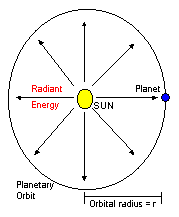### PLANETARY TEMPERATURES

Planets have surface temperatures above absolute zero because they exist in the solar system, at the heart of which is the sun which radiates energy from its surface at the rate of:
Ls = 3.86x1026 Watts (or Joules per second)
We now need to consider how much of this energy is intercepted by a planet of radius R at a distance r from the Sun.Consider the hypothetical sphere surrounding the Sun with a radius r and centred on the Sun. The radius of this sphere is the same as the radius of the planetary orbit.

Now all the energy radiated by the Sun has to pass through this hypothetical sphere, which has a surface area of 4 p r2 . Thus the amount of solar energy that passes through an area of one square metre of the sphere is given by the formula:

S = Ls / ( 4 p r2 )

where S is termed the solar constant for that planet. The table below lists the results from this formula for the four terrestrial planets Mercury, Venus, Earth and Mars.

Solar Constants
 Mercury 9159 W/m2 Venus 2623 W/m2 Earth 1373 W/m2 Mars 591 W/m2

The total energy that is intercepted by the planet is the solar constant times the projected area that the planet presents to the solar radiation. This is essentially the area of the planetary disc which is p R2. Thus the energy input to the planet is given by the formula:

Ein = S p R2

Now this energy input is balanced by the energy that the planet radiates back out into space. The energy radiated by an ideal (black-body radiator) planet with no atmosphere is given by the Stefan-Boltzmann equation:

Eout = s A T4

where A is the area of the planet that contributes to the reradiation of the energy, and T is the mean temperature of that surface area. If the planet is not rotating, then the only area that radiates any significant energy is the hemisphere that faces the sun. However, if the planet rotates with a reasonably short period, then the energy received from the sun will be distributed over the entire surface area of the planet, and the entire globe will thus participate in the reradiation of energy back out into space. This area is given by A = 4 p R2. By equating the energy radiated out into space to the input energy received from the sun, we can obtain an expression for the mean temperature of the planetary surface. This temperature is given by:

T = { Ls / ( 16 p s r2 ) }1/4

It is interesting to note that this temperature depends only upon the distance of the planet from the Sun and not upon the size of the planet. The table below lists the mean planetary temperatures expected for the four terrestrial planets if they had no atmosphere, were ideal radiators and were rotating with a period measured in no more than a few tens of hours.

Computed Mean Planetary Temperatures
 Mercury 448 K (175 C) Venus 328 K (55 C) Earth 279 K ( 6 C) Mars 226 K (-47 C)

Deviations of actual planetary surface temperatures from these computed temperatures are due to:
• Presence of an atmosphere
However, the computed temperatures give us a baseline to indicate what we need to do, or rather what it may be physically possible to do to change that planet's surface temperature.

Thus for humans, the ideal Martian temperature is much colder than what we would like, and so we must look to implementing a substantial greenhouse effect via an atmosphere.

For Venus (disregarding the actual temperatures at present), we need to increase the albedo (reflectivity) of the planet from the ideal to reduce the surface temperature.

The Earth already has a very slight greenhouse effect through the existence of atmospheric greenhouse gases, and thus its mean surface temperature is just slightly higher than the ideal temperature we have computed above.

The actual mean surface temperatures of Venus, Earth and Mars are given below.

Actual Mean Planetary Termperatures
 Venus 740 K (467 C) Earth 288 K (15 C) Mars 220 K (-53 C)

Thus we see that both Earth and Mars are reasonably close to their ideal temperatures, with Earth being slightly higher and Mars slightly lower than that predicted for a planet with no atmosphere. We might thus be tempted to conclude that the atmosphere of both these planets has only a second order effect on the surface temperature.

In the case of Venus however, the surface temperature is substantially higher than what our ideal equations predicted, and indeed the very thick Venusian atmosphere has a controlling effect on the surface temperature of this planet. To reduce the temperature of Venus we thus either need to get rid of a substantial fraction of the atmosphere of we need to change its composition.